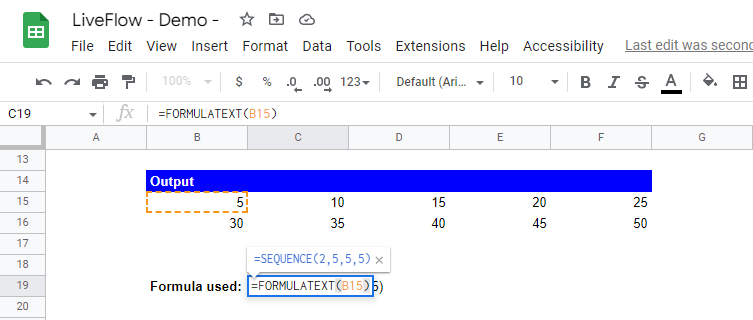# SEQUENCE Function in Google Sheets: Explained

## How does the SEQUENCE formula work in Google Sheets?

The SEQUENCE function in Google Sheets is a function that generates a sequence of numbers in the specified range based on a starting value and a step value. The SEQUENCE can be helpful in various situations where you want to generate a series of numbers with a particular pattern in Google Sheets.

## How do you use the SEQUENCE function in Google Sheets?

Here is the general syntax for the SEQUENCE function:

``=SEQUENCE(rows, [columns], [start], [step])``

rows: The number of rows in the array.

columns [Optional]: The number of columns in the array. If not specified, the default is 1.

start [Optional]: The starting value for the sequence. If not specified, the default is 1.

step [Optional]: The increment between each value in the sequence. If not specified, the default is 1.

For example, if you wanted to generate a sequence of numbers from 1 to 10, you could use the following formula:

``=SEQUENCE(10)``

This would generate a 1x10 array of numbers from 1 to 10, like this:

You can also use the other three parameters, columns, start, and step arguments to customize the sequence. For example, if you wanted to generate a 2x5 array of numbers starting from 5, with a step value of 5, you could use the following formula:

``=SEQUENCE(2, 5, 5, 5)``

This would generate a 2x5 array of numbers from 5 to 50, with a step value of 5, like this:How to develop a 2x5-sized array starting from 5 with a step-up of 5 in Google Sheets

Learn how to do this step-by-step in the video below 👇

## Need help?

Our team is here to help you any time between 9am and 10pm EST.Email us at: help@liveflow.io